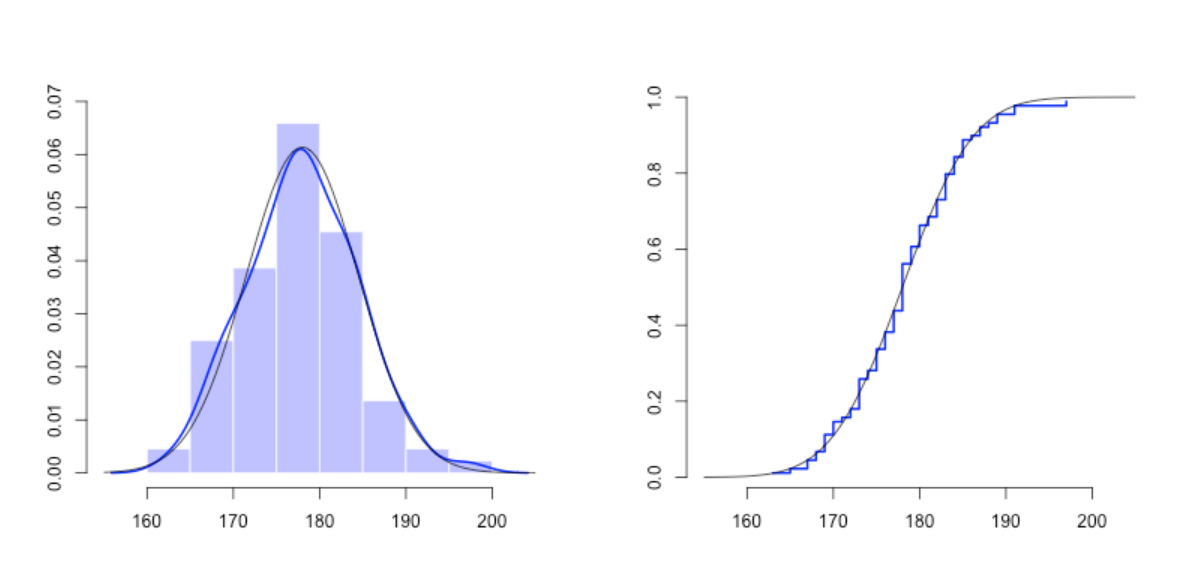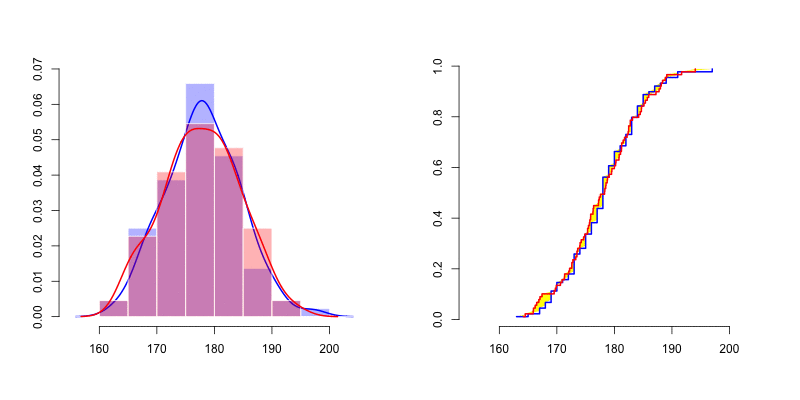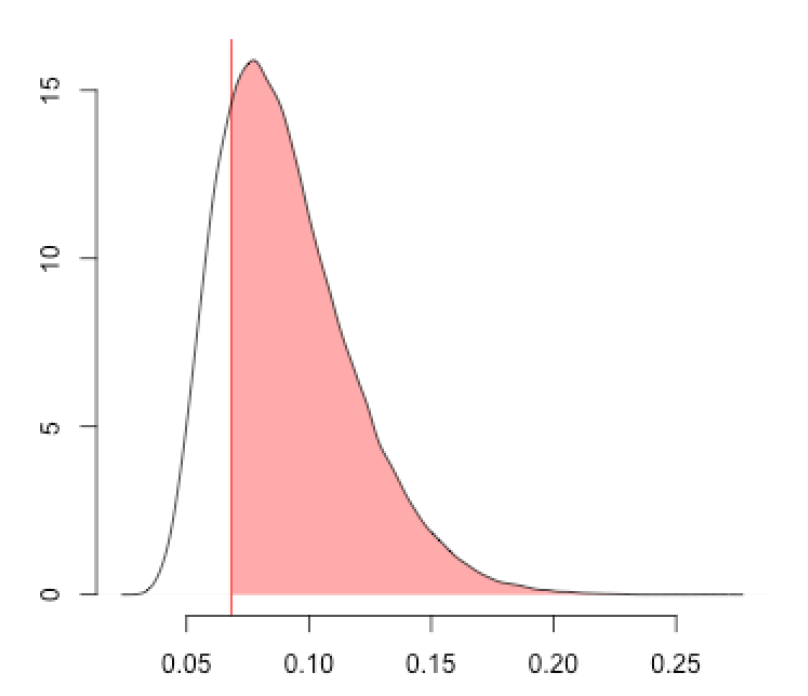# R语言使用蒙特卡洛模拟进行正态性检验及可视化

``X=Davis\$height[Davis\$sex=="M"]``

``````u=seq(155,205,by=.5)
par(mfrow=c(1,2))
hist(X,col=rgb(0,0,1,.3))
lines(density(X),col="blue",lwd=2)
lines(u,dnorm(u,178,6.5),col="black")
Xs=sort(X)
n=length(X)
p=(1:n)/(n+1)
plot(Xs,p,type="s",col="blue")
lines(u,pnorm(u,178,6.5),col="black")````````````mean(dks)
 0.78248``````

``````ks.test(X,"pnorm",178,6.5)

One-sample Kolmogorov-Smirnov test

data:  X
D = 0.068182, p-value = 0.8079
alternative hypothesis: two-sided``````

Kaizong Ye拓端研究室（TRL）的研究员。

​非常感谢您阅读本文，如需帮助请联系我们！QQ在线咨询

15121130882

0571-63341498

## 关注有关新文章的微信公众号

This will close in 0 seconds